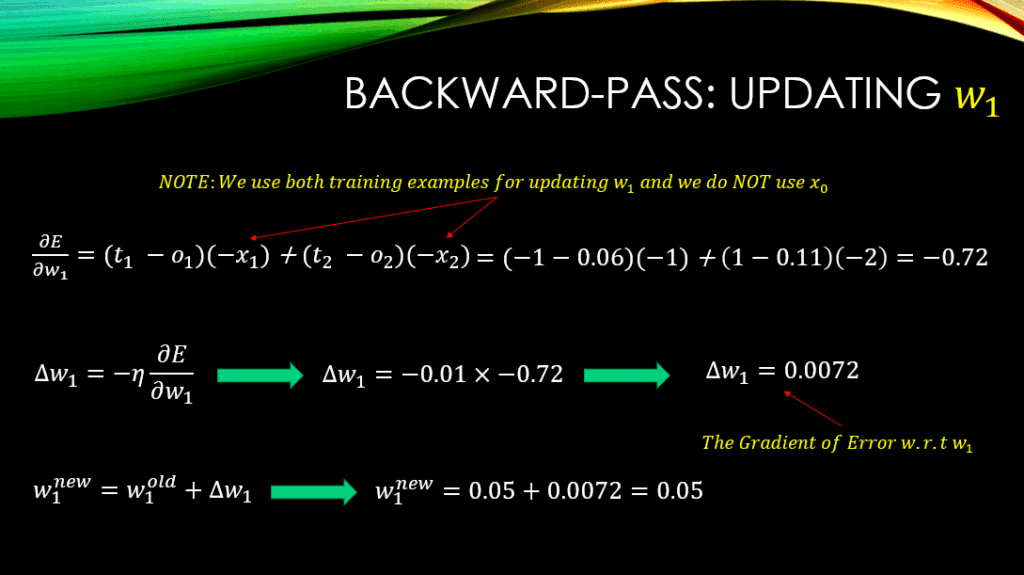## What Will You Learn?

In our previous post, we have talked about the meaning of gradient descent and how it can help us update the parameters of our Artificial Neural Network (ANN). In this post we will actually mathematically derive the update rule using the concept of gradient descent. We will also look at a NUMERICAL example and literally update the weights of a neural network by hand, to make sure we understand the concept. So, by the end of this post, you will have a good understanding of how to derive the update rule for the parameters of your network, given an error function of choice, using the concept of gradient descent that we have discussed in our previous post. I am ready when you are 😉

## Let's derive the Update Rule

As you remember, we said that the update rule for a given weight,, (generally speaking) is:

where:

######And we emphasized thatis called the gradient of our error,, with respect to our parameter,.  Finally, we talked aboutwhich is our learning rate (a.k.a., the step size). Now it is time to consider a quite simple neural network, with some weights to be learned, and an error function of choice to be minimized. Consider the simple neural network below: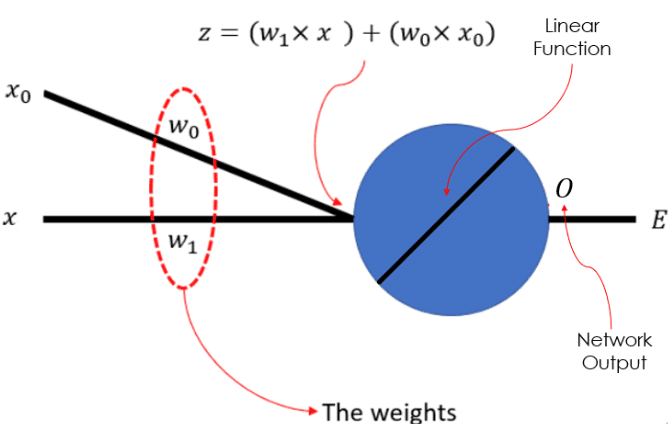Figure 1: A Simple Neural Network

In this network, we have a 1-dimensional input data,, with a bias unit,, that is always equal 1, by definition. We have 2  sets of weightsand, which we are trying to learn using gradient descent. The actual big blue circle is a linear neuron andrepresents the output of the neural network. As you can see, the input data and the weights are linearly combined to make, which is also called the pre-activation of the neuron. We will further define an error function,, that we are trying to minimize by learning an appropriate set of values for our weights. Let’s define an error function:You notice that this error is a function of the weight vector, which is a vector of all the weights in our neural network to be learned. So, for every input training data, in our training set, we generate the output of our model, that is. Then we compute the squared difference between this output and the desired output. We compute this squared difference for every input data, and sum them all up and finally divide it by 2, to compute the total error across the entire training set. So, you can see that minimizing this error across the entire training set, means that for every input data, the output of our modelis getting quite close to the target value, which our ground-truth.

### One a side note, there is a very good reason why we have the square operation and the division by 2, in this definition of error. I have explained this in details in my course “The Birth of Error Functions in Neural Networks”. Click here to enroll (It is Free!)

###### So, now we need to take the derivative of our error function with respect to every one of the weights in our network. This will be the gradient of the error with respect to the weights. As a result, for every weightin our network, the derivative of the errorwith respect to that weight, which is denoted as, is computed as follows: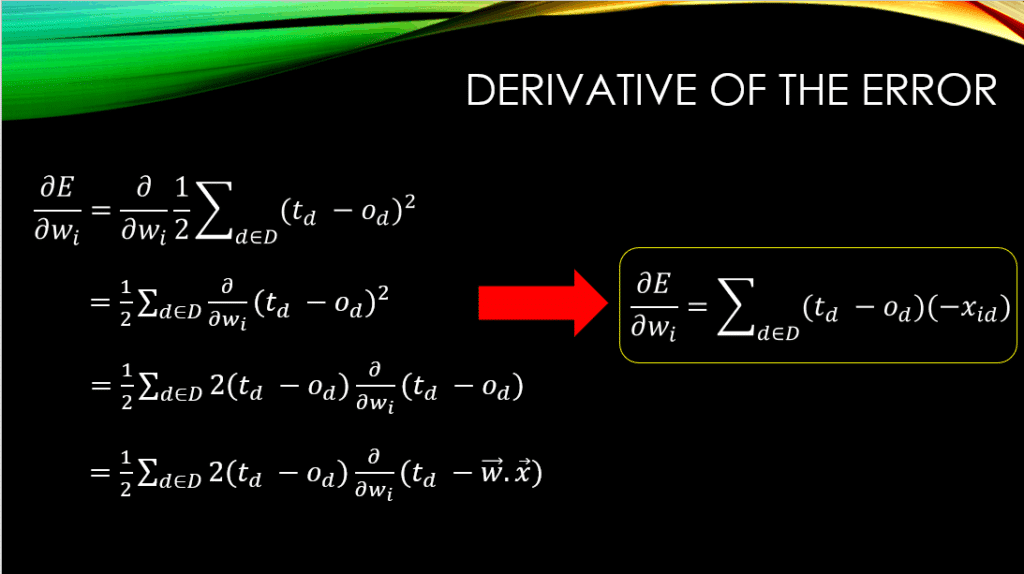Figure 2: Deriving the Derivative of the Error w.r.t the Weights

Please note that, means thedimension of thetraining example.

In other words, if a weight connected to thedimension of the input,, then that weight can only be effected by that dimension of the input, and NOT the other dimensions! This is why when computing the gradient w.r.twe are only considering thedimension of the input, in the derivations above.

Now we have the gradient and it is time to incorporate that in, to measure the amount by which we need to change every weight: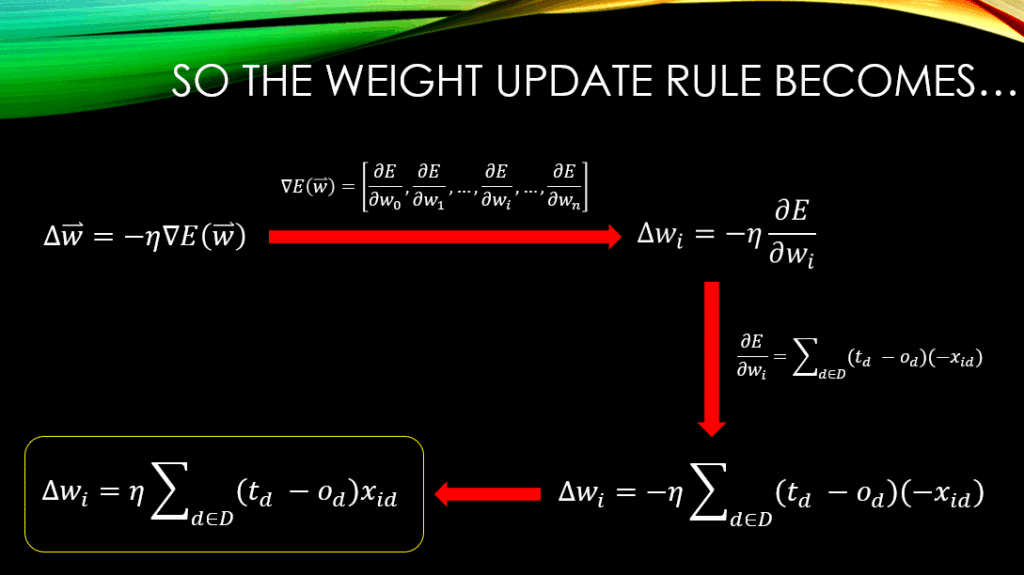Figure 3: Deriving the Actual Change to be Applied to the Weights

So, what this means is that by initializing our weights randomly, we will keep them fixed and pass the entire training set through our neural network and generate the outputs for each of the training examples. Then we will compute the error across all of these outputs using our error function and ground-truth labels. For a given learning rate,, the computedtells us how much we need to change,, in order to minimize the total error,, the fastest, as discussed in our previous post on Gradient Descent. Finally, we will add this to the current value of our weight in order to update that weight, using the learning rule:

## A Numerical Example for the Whole Process

###### I am a big fan of simplicity, so let’s give a super simple example. We will define a training set of 2 training data (I know, too small but it is easier to understand). The neural network is the same that is depicted in Fig.1. In this neural network, please note thatis called the bias unit and it is always equal to 1, that is to say. As a result the only input from which we will be able to feed in our training data into the neural network would be through. This means that our training data are 1-dimensional. Moreover, we will have to initialize our 2 weights randomly as well and define a learning rate. Finally, in our training set, for every training data, we will also have a ground-truth, so that we could actually compute the error and update the weights in the network. So, all of these are defined as follows:
1. Training Data:=1 and=2
2. The Ground Truth:=-1 and=1
3. The weights of the neural network are initialized randomly:=0.01 and=0.05
4. Finally let’s set the learning rate:=0.01
###### Now we will input every data point into the network according to Fig.1, compute the outputs, and compute the error for each of the outputs. This is called the forward-pass phase where the data travels from the input’s side to the output’s side of a neural network.

In all of the following derivations,is thetraining example. And as always,is the bias unit that is always equal to 1! Finally,,, and, represent the output, ground-truth, and error value for theinput training data.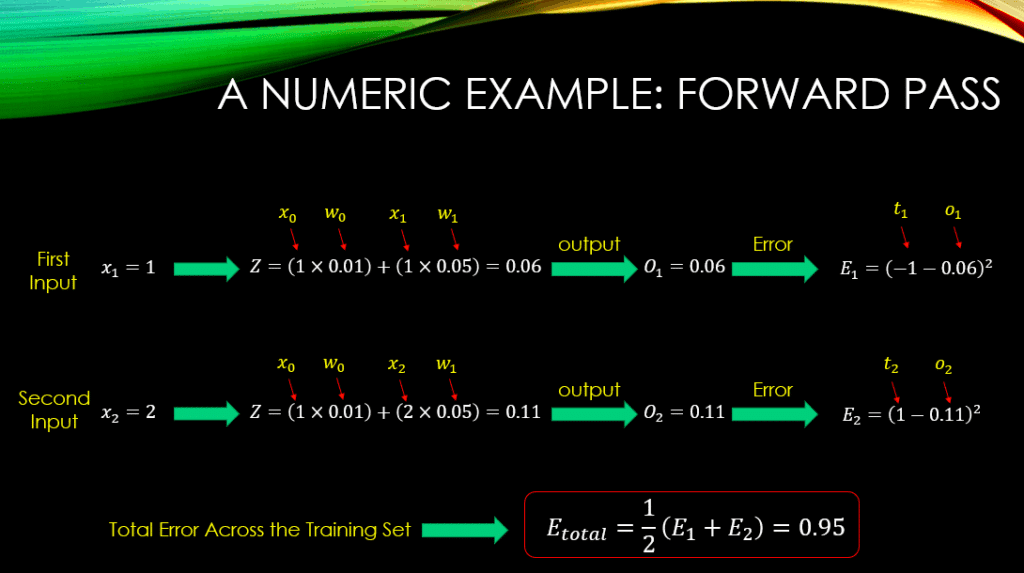Figure 4: The forward-pass for Computing the Total Error Across the Training Set

###### Finally we will add this value to the old value of our weights, to compute their new values. This is called LEARNING! We are learning the weights based on the errors that we make for every training data! Now, let’s update: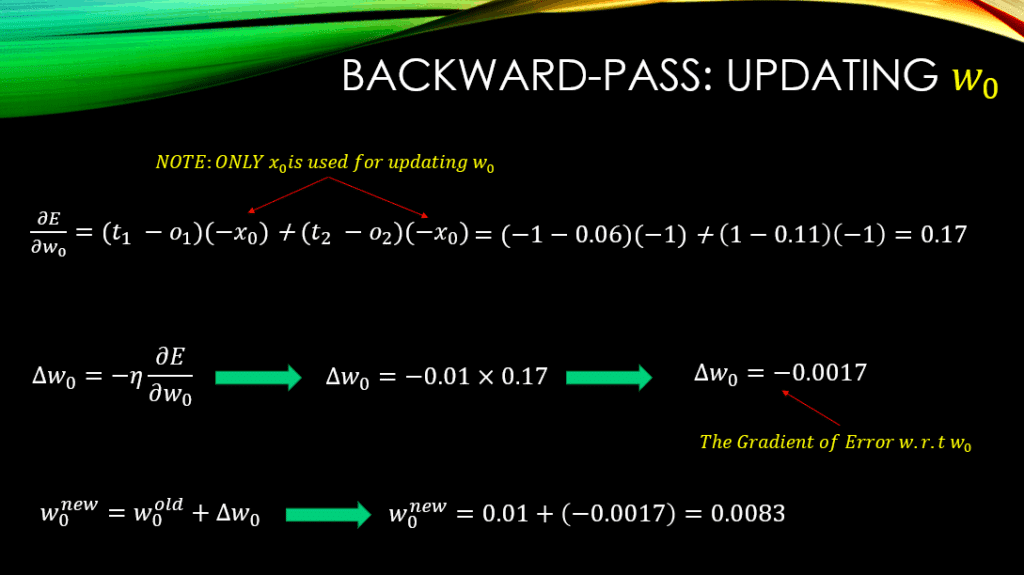###### You note that the only inputs that we have used for computing the gradients in here, is the bias unit and NONE of the training examples in our training set. Why? Because that is the ONLY input that is connected toand the actual training data that are being inputted to the neural network are connected toand NOT! So, now we have a new value for our! Now, let’s update: